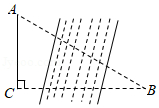$\text{A.}$ $2 \mathrm{~km}$ $\text{B.}$ $3 \mathrm{~km}$ $\text{C.}$ $2 \sqrt{3} \mathrm{~km}$ $\text{D.}$ $4 \mathrm{~km}$

D

#### 解析：

\begin{aligned} &\therefore \angle B=30^{\circ}, \\ &\therefore A B=2 A C=4(\mathrm{~km}) . \end{aligned}

①点击 首页查看更多试卷和试题 , 点击查看 本题所在试卷